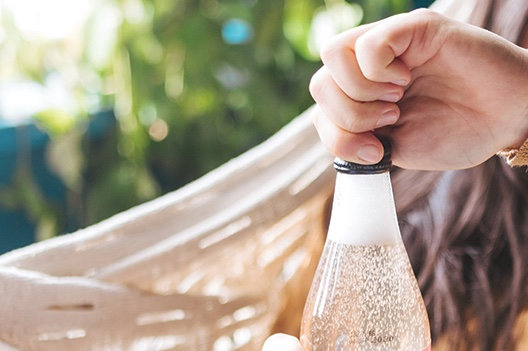# Opening bottle with sparkling water#

Written by Svetlana Kyas (ETH Zurich) on Mar 31th, 2022

This tutorial shows how to simulate the solubility of CO2 in water or, more simply, the effect of the carbon dioxide released when you open the bottle of sparkling water.Opening bottle with sparkling water, Source: flavorman.com

First, we define the chemical system:

from reaktoro import *

db = SupcrtDatabase("supcrtbl")

# Create an aqueous phase automatically selecting all species with provided elements
aqueousphase = AqueousPhase(speciate("H O C"))
aqueousphase.setActivityModel(chain(
ActivityModelHKF(),
ActivityModelDrummond("CO2"),
))

# Create a gaseous phase
gaseousphase = GaseousPhase("CO2(g)")
gaseousphase.setActivityModel(ActivityModelPengRobinson())

# Create the chemical system
system = ChemicalSystem(db, aqueousphase, gaseousphase)

# Create the equilibrium solver
solver = EquilibriumSolver(system)


Then, we define the range of pressures using the linspace() function of the numpy library. The initial and final pressures correspond to the values in the bubble bottle before and after opening.

import numpy as np
closedP = 3.79 # in bars
openP = 1.01325 # in bars
pressures = np.linspace(openP, closedP, num=100)


Note: A typical carbonated soft drink contains approximately 3–4 volumes (6–8 g/L) CO2. To obtain the amount of mol of CO2, we need to perform the following calculations: 8 g/L = 8 / 44.01 mol = 0.18 mol, where 44.01 g/mol is the CO2 molar mass.

Next, we go through the created pressure list and collect the CO2(g) amounts obtained in the equilibrated chemical for a given pressure.

import pandas as pd
df = pd.DataFrame(columns=["P", "amountCO2"])

for P in pressures:
state = ChemicalState(system)
state.setTemperature(20.0, "celsius")
state.setPressure(P, "bar")

res = solver.solve(state)

df.loc[len(df)] = [P, float(state.speciesAmount("CO2(g)"))]


To visualize the changes in the CO2(g) amount in the bottle, we export bokeh python plotting package.

from bokeh.plotting import figure, show
from bokeh.models import HoverTool
from bokeh.io import output_notebook
output_notebook()

hovertool = HoverTool()
hovertool.tooltips = [("amount(CO2) in brine", "@amountCO2 mol"),
("P", "@P")]

p = figure(
title="CO2(G) AMOUNT IN SPARKLING WATER BOTTLE BEFORE AND AFTER OPENING",
x_axis_label=r'PRESSURE [BAR]',
y_axis_label='AMOUNT OF CO2(G) [MOL]',
sizing_mode="scale_width",
plot_height=300)